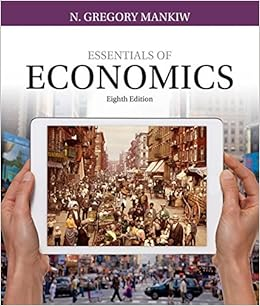# A firm increased its production and sales because the...

• Homework Help
• 4
• 83% (24) 20 out of 24 people found this document helpful

This preview shows page 1 - 2 out of 4 pages.

HW7

1 )   A firm increased its production and sales because the firm 's manager rearranged the layout of his factory floor . This is an example of
A ) investment in human capital .
B ) economies of scale .
C ) positive technological change .
D ) inspired management .
Answer:  D )   positive technological change .
2 )   The difference between technology and technological change is that
3 )   Which of the following is a factor of production that generally is fixed in the short run ?
4 )   Which of the following is the best example of a short - run adjustment ?
5 )   The production function shows
A ) the total cost of producing a given quantity of output .
B ) the maximum output that can be produced from each possible quantity of inputs .
C ) the technology used to produce output .
D ) the incremental output gained by improving the production process .
Answer:  C )   the maximum output that can be produced from each possible quantity of inputs .
6 )   Which of the following statements best describes the economic short run ?
7 )   Which of the following statements is true ?
8 )   The marginal product of labor is defined as
9 )   Red Stone Creamery currently hires 5 workers . When it added a 6th worker , its output actually fell . Which of the following statements is true ?
A ) The marginal product of the sixth worker must be negative .
B ) The average product of the sixth worker is negative .
C ) The sixth worker is not as skilled as the fifth worker .
D ) The total product becomes negative .
Answer:  a.   The marginal product of the sixth worker must be negative .
10 )   The law of diminishing marginal returns
11 )   As a firm hires more labor in the short run , the
12 )   Which of the following explains why the marginal cost curve has a U shape ?
##### We have textbook solutions for you!
The document you are viewing contains questions related to this textbook.The document you are viewing contains questions related to this textbook.
Chapter 12 / Exercise 03
Essentials of Economics
MankiwExpert Verified
Homework 7 Chapter 11 MULTIPLE CHOICE. Choose the one alternative that best completes the statement or answers the question. 1) A firm increased its production and sales because the firm's manager rearranged the layout of his factory floor. This is an example of A) investment in human capital. B) economies of scale. C) positive technological change. D) inspired management.
2) The difference between technology and technological change is that
3) Which of the following is a factor of production that generally is fixed in the short run?
4) Which of the following is the best example of a short-run adjustment?
##### We have textbook solutions for you!
The document you are viewing contains questions related to this textbook.The document you are viewing contains questions related to this textbook.
Chapter 12 / Exercise 03
Essentials of Economics
MankiwExpert Verified
•••